JEE  >  VITEEE PCME Mock Test - 1

# VITEEE PCME Mock Test - 1

Test Description

## 125 Questions MCQ Test VITEEE: Subject Wise and Full Length MOCK Tests | VITEEE PCME Mock Test - 1

VITEEE PCME Mock Test - 1 for JEE 2023 is part of VITEEE: Subject Wise and Full Length MOCK Tests preparation. The VITEEE PCME Mock Test - 1 questions and answers have been prepared according to the JEE exam syllabus.The VITEEE PCME Mock Test - 1 MCQs are made for JEE 2023 Exam. Find important definitions, questions, notes, meanings, examples, exercises, MCQs and online tests for VITEEE PCME Mock Test - 1 below.
Solutions of VITEEE PCME Mock Test - 1 questions in English are available as part of our VITEEE: Subject Wise and Full Length MOCK Tests for JEE & VITEEE PCME Mock Test - 1 solutions in Hindi for VITEEE: Subject Wise and Full Length MOCK Tests course. Download more important topics, notes, lectures and mock test series for JEE Exam by signing up for free. Attempt VITEEE PCME Mock Test - 1 | 125 questions in 150 minutes | Mock test for JEE preparation | Free important questions MCQ to study VITEEE: Subject Wise and Full Length MOCK Tests for JEE Exam | Download free PDF with solutions
 1 Crore+ students have signed up on EduRev. Have you?
VITEEE PCME Mock Test - 1 - Question 1

### The area between the curve $y=1-|x|$ and x-axis is

Detailed Solution for VITEEE PCME Mock Test - 1 - Question 1 Plot the graph of 1-|x| to plot it first you know graph of |x| then taking x-axis as the mirror take image of |x| then shift the the graph 1 unit upward that will be your final graph the 3 vertices of the triangle so formed will be (-1,0) ,(0,1), (1,0) then find the area it will be 1.
VITEEE PCME Mock Test - 1 - Question 2

### For the curve x = t2 - 1, y = t2 - t tangent is parallel to x-axis where

VITEEE PCME Mock Test - 1 - Question 3

### If ω is a complex cube root of unity ,then the value of ω 99 + ω 100 + ω101 is

Detailed Solution for VITEEE PCME Mock Test - 1 - Question 3 1+w+w²=0
divide w powers by 3 and put remainders as powers then you will get 0
VITEEE PCME Mock Test - 1 - Question 4

If |(z-1)/(z-i)| = 1, then the locus of z is

VITEEE PCME Mock Test - 1 - Question 5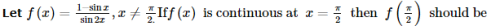Detailed Solution for VITEEE PCME Mock Test - 1 - Question 5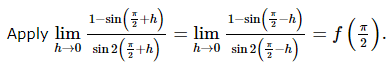VITEEE PCME Mock Test - 1 - Question 6

The differential equation representing the family of curves y2=2c(x+√c), where c is a positive perimeter, is of

VITEEE PCME Mock Test - 1 - Question 7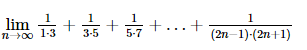VITEEE PCME Mock Test - 1 - Question 8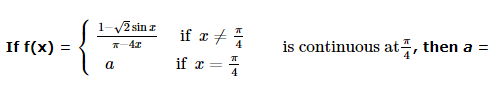VITEEE PCME Mock Test - 1 - Question 9

If a, b, c are different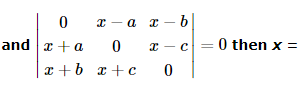VITEEE PCME Mock Test - 1 - Question 10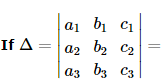and A1 , B1 , C1  etc are cofactors of a 1 , b 1 , c 1 , then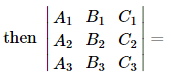VITEEE PCME Mock Test - 1 - Question 11
The solution of the equation (2y-1) dx-(2x+3) dy=0 is
VITEEE PCME Mock Test - 1 - Question 12

The solution of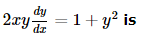is

Detailed Solution for VITEEE PCME Mock Test - 1 - Question 12 2xydy/dx = 1 + y²

2 ydy/1 + y² = dx/x

Integrating

log(1 + y²) = logx + c

log(1 + y²/x) = c

1 + y²/x = e^c(new constant) = c′

1 + y² = cx
VITEEE PCME Mock Test - 1 - Question 13

Differential of x6 w.r. to x3 is equal to

Detailed Solution for VITEEE PCME Mock Test - 1 - Question 13 Let
y = x^6
z = x³

To find : dy/dz

dy/dx = 6x^5

dz/dx = 3x²

dy/dx × dx/dz = 6x^5/3x²

= 2x³
VITEEE PCME Mock Test - 1 - Question 14

If c is parameter then the differential equation whose solution is y = c2 + c/x is

Detailed Solution for VITEEE PCME Mock Test - 1 - Question 14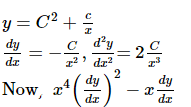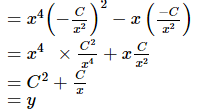VITEEE PCME Mock Test - 1 - Question 15

The minimum value of sinx + cosx is

VITEEE PCME Mock Test - 1 - Question 16

What is the value of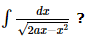VITEEE PCME Mock Test - 1 - Question 17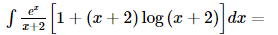VITEEE PCME Mock Test - 1 - Question 18

If cot-1[(cos α)1/2] - tan-1[(cot α)1/2] = x, then sin x =

VITEEE PCME Mock Test - 1 - Question 19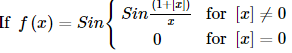where [x] denotes the greatest integer not exceeding x, then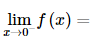VITEEE PCME Mock Test - 1 - Question 20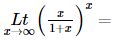VITEEE PCME Mock Test - 1 - Question 21

Which statement represents the inverse of the statement "If it is snowing, then Skeeter wears a sweater."?

Detailed Solution for VITEEE PCME Mock Test - 1 - Question 21

To form the INVERSE of a statement, insert "NOT" into the "If" and the "then" sections of the sentence.

VITEEE PCME Mock Test - 1 - Question 22

A and B are square matrices of the same order. Which is correct?

VITEEE PCME Mock Test - 1 - Question 23

If |A| represents the determinant of a square matrix of order 3 then (-2A)=

VITEEE PCME Mock Test - 1 - Question 24

From a box containing 10 cards, numbered 1, 2,3,......,10. Four cards are drawn together, what is the probability of their sum is even ?

VITEEE PCME Mock Test - 1 - Question 25

The number of ways in which a committee of 6 members can be formed from 8 gentlemen and 4 ladies so that the committee contains atleast 3 ladies is

Detailed Solution for VITEEE PCME Mock Test - 1 - Question 25

A committee of 6 members is to formed from 8 gentlemen and 4 ladies by taking
(i) 3 lady out of 4 and 3 gentlemen out of 8
(ii) 4 lady out of 4 and 2 gentlemen out of 8
In case (i) the number of ways = 4c3 x 8c3
= 4 x 56 = 224
In case (ii) the number of ways = 4c4 x 8c2
= 1 x 28 = 28
Hence, the required number of ways = 224 + 28 = 252

VITEEE PCME Mock Test - 1 - Question 26

The probabilites of solving a problem by three students A, B, C independently are 1/3 , 1/4 , 1/5 . The probability that the problem will be solved is

VITEEE PCME Mock Test - 1 - Question 27

If A and B are two mutually exclusive events such that P(B) = 2P(A) and A ∪ B = S. Then P(A) is equal to

VITEEE PCME Mock Test - 1 - Question 28

The harmonic mean of the roots of the equation (5 + √2)x2 - (4 + √5)s + 8 + 2√5 = 0 is

VITEEE PCME Mock Test - 1 - Question 29

The average of values 0, 2, 4, 6, 8, ...., 2n is

VITEEE PCME Mock Test - 1 - Question 30

In ΔABC, if cot A/2 = (b + c)/a , then the triangle is

Detailed Solution for VITEEE PCME Mock Test - 1 - Question 30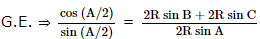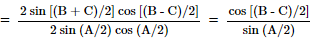⇒ cos (A/2) = cos [(B - C)/2]
⇒ A/2 = (B - C)/2
⇒ A = B - C
⇒ A + C = B
⇒ B = 900.

VITEEE PCME Mock Test - 1 - Question 31

Two numbers within the brackets denote the ranks of 10 students of a class in two subjects - (1, 10), (2, 9), (3, 8), (4, 7), (5, 6), (6, 5), (7, 4), (8, 3), (9, 2), (10, 1), then rank correlation coefficient is

VITEEE PCME Mock Test - 1 - Question 32

If the roots of the equation x2+2mx+m-2m+6=0 are equal, then the value of m is

VITEEE PCME Mock Test - 1 - Question 33

If there is a multiple root of order 3 for the equation x4 − 2x3 + 2 x − a = 0 , then the other roots is

VITEEE PCME Mock Test - 1 - Question 34
The ratio in which the line joining (2,4,5), (3,5,-9) is divided by the YZ-plane is
VITEEE PCME Mock Test - 1 - Question 35

The line (p + 2q) x + (p - 3q) y = p - q for different values of p and q passes through the fixed point

VITEEE PCME Mock Test - 1 - Question 36

In a three dimensional space, the equation 3x - 4y = 0 represents

VITEEE PCME Mock Test - 1 - Question 37

If the pair of lines ax2 + 2hxy + by2 + 2gx + 2fy + c = 0 intersect on the y-axis then

VITEEE PCME Mock Test - 1 - Question 38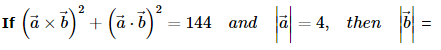VITEEE PCME Mock Test - 1 - Question 39

If sin(x-y) = cos(x+y) = 1/2, the value of x and y lying between 00 and 1800, are given by

Detailed Solution for VITEEE PCME Mock Test - 1 - Question 39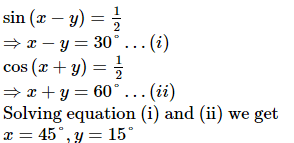VITEEE PCME Mock Test - 1 - Question 40

If A(-1,2,3),B(1,1,1) and C(2,-1,3) are points in a plane, the unit vector perpendicular to the plane AB is

VITEEE PCME Mock Test - 1 - Question 41

In a transformer, current and e.m.f. in the output is 10 A and is 220 V respectively. If ratio of no. of turns in secondary and primary coil 50:10, then current in the primary coil is

VITEEE PCME Mock Test - 1 - Question 42

The closest distance of approach of an α - particle travelling with a velocity 'V' to a certain nucleus is 'x'. The distance of closest approach of α - particle travelling with a velocity 3V to the same nucleus is

Detailed Solution for VITEEE PCME Mock Test - 1 - Question 42 At closest approach K.E = P.E
I.e. 1/2mv2= 1/4π€0×( Ze×2e)/r here r is distance of closest approach
r is inversely proportional to square of velocity
r1/r2=(v2/v1)^2=(3v/v)^2=9
X/r2=9. r2=X/9
VITEEE PCME Mock Test - 1 - Question 43

A charged particale accelerated through a P.D. of 100 volt passes throught the uniform electric and magnetic fields so as to experience no deflection. If E = 15 x 106 V m-1 and B = 5 x103 T , then the specific charge of the particle is

VITEEE PCME Mock Test - 1 - Question 44

A parallel plate capacitor of plate area A and the plate separation d is charged to potential difference V and then the battery is disconnected. A slab of dielectric constant K is then inserted between the plates of the capacitor so as to fill the space between the plates. If Q, E and W denote respectively, the magnitude of charge on each plate, the electric field between the plates and work done by the system in question, in the process of inserting the slab, then select the incorrect relation from the following :

VITEEE PCME Mock Test - 1 - Question 45

The relation between Faraday constant F, electron charge e, and Avogardo Number N is

VITEEE PCME Mock Test - 1 - Question 46

Consider telecommunication through optical fibres. Which of the following statements is not correct

VITEEE PCME Mock Test - 1 - Question 47

Two wires of same material have length L and 2L and cross-sectional areas 4A and A respectively. The ratio of their specific resistance would be

VITEEE PCME Mock Test - 1 - Question 48

Two non-ideal batteries are connected in parallel. Consider the following statements.
(i)The equivalent emf is smaller than either of the two emfs.
(ii)The equivalent internal resistance is smaller than either of the two internal resistances.

VITEEE PCME Mock Test - 1 - Question 49

There are three voltmeters of the same range but of resistances 10000 Ω, 8000 Ω and 4000 Ω respectively. The best voltmeter among these is the one whose resistance is

VITEEE PCME Mock Test - 1 - Question 50

Quantum theory of light gives concept of

VITEEE PCME Mock Test - 1 - Question 51

Choke coil works on the principle of

VITEEE PCME Mock Test - 1 - Question 52

In a H - atom an electron makes 6.6x1015  rev/sec around the nucleus. Then its equivalent current is

VITEEE PCME Mock Test - 1 - Question 53

A charge q is placed at the corner of a cube of side l. The electric flux passing through the cube is

Detailed Solution for VITEEE PCME Mock Test - 1 - Question 53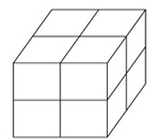Eight cubes, each side l, are required to form a Gaussian surface
So that the charge q at the corner of a small cube appears at the centre of the bigger cube
According to Gauss's law, the electric flux through the bigger cubes = q/∈0
Hence the electric flux through the given small cube = q/8∈0

VITEEE PCME Mock Test - 1 - Question 54

The core of transformer is laminated to reduce the effect of

VITEEE PCME Mock Test - 1 - Question 55

VITEEE PCME Mock Test - 1 - Question 56

On moving a charge of 20 C by 2 cm, 2 joule of work is done, then the potential difference between the points is

Detailed Solution for VITEEE PCME Mock Test - 1 - Question 56

W = QV
∴ V = W/Q = 2/20 = 0.1 volt

VITEEE PCME Mock Test - 1 - Question 57

A loop of area 0.1 m2 rotates with a speed of 60 rps perpendicualr to a magnetic field of 0.4 T. If there are 100 turns in the loop, maximum voltage induced in the loop is

VITEEE PCME Mock Test - 1 - Question 58

The time in seconds required to produce a potential diference of 20V across a capacitor of 1000 μ F when it is charged at the steady rate of 200 μ C/s is

VITEEE PCME Mock Test - 1 - Question 59

Equal charges are given to two conducting spheres of different radii. The potential will

VITEEE PCME Mock Test - 1 - Question 60

Two point charges +q and -q are held fixed at (-d,0) and (d,0) respectively of a x-y coordinate system. Then

VITEEE PCME Mock Test - 1 - Question 61

When two deuterium nuclei fuse together, in addition to tritium, we get a

VITEEE PCME Mock Test - 1 - Question 62

Current is flowing in two long parallel conductors in the same direction. The conductors

VITEEE PCME Mock Test - 1 - Question 63

The radius of an electron orbit in a hydrogen atom is of the order of

VITEEE PCME Mock Test - 1 - Question 64

The decay constant of a radio active substance is λ . Its half life is

VITEEE PCME Mock Test - 1 - Question 65

Stopping potential for photoelectrons

VITEEE PCME Mock Test - 1 - Question 66

A parallel plate air condenser has certain capacity. When 2/3 of the distance between the plates is filled with a dielectric, the capacity is found to increase by a factor of 2.25. The dielectric constant is

Detailed Solution for VITEEE PCME Mock Test - 1 - Question 66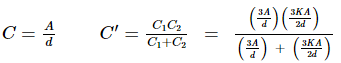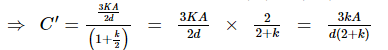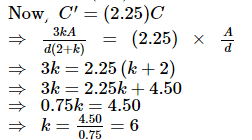VITEEE PCME Mock Test - 1 - Question 67

Two radiations containing photons of energy twice and five times the work function of the metal are incident successively on the metal surface. The ratio of the maximum velocities of the emitted electrons in the two cases will be

VITEEE PCME Mock Test - 1 - Question 68

The far point of a myopic eye is 1.5 m. To correct this defect of the eye, the power of lens is

VITEEE PCME Mock Test - 1 - Question 69

A plano convex lens is made refractive index 1.6. The radius of curvature of the curved surface is 60 cm. The focal length of the lens is

VITEEE PCME Mock Test - 1 - Question 70

The function of base layer in a transistor is similar to that --- in a vacuum triode.

VITEEE PCME Mock Test - 1 - Question 71

The electric circuit used to get smooth d.c. output from a rectifier circuit is called

VITEEE PCME Mock Test - 1 - Question 72

At 0 K intrinsic semiconductors behaves as

VITEEE PCME Mock Test - 1 - Question 73

The ratio of work function and temperatures of two emitters are 1:2, then the ratio of current densities obtained by them will be

VITEEE PCME Mock Test - 1 - Question 74

P-N junction is said to be forward biased, when

VITEEE PCME Mock Test - 1 - Question 75

In intrinsic semiconductor at room temperature, number of electrons and holes are

VITEEE PCME Mock Test - 1 - Question 76

Ga As is

VITEEE PCME Mock Test - 1 - Question 77

Two waves of intensities I and 4 I superimpose, then maximum and minimum intensities are

Detailed Solution for VITEEE PCME Mock Test - 1 - Question 77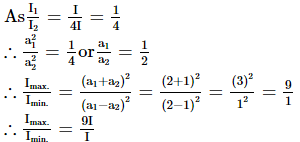VITEEE PCME Mock Test - 1 - Question 78

If a star is moving towards earth, then the lines are shifted towards

VITEEE PCME Mock Test - 1 - Question 79

In Huygen's wave theory, the locus of all points oscillating in the same phase is called a

VITEEE PCME Mock Test - 1 - Question 80

A wave front travels

VITEEE PCME Mock Test - 1 - Question 81

Rectified spirit is a mixture of

VITEEE PCME Mock Test - 1 - Question 82

Carbonyl compounds undergo nucleophilic addition because of

Detailed Solution for VITEEE PCME Mock Test - 1 - Question 82 Carbonyl carbon acquires positive charge,thus nucleophile can attack on it easily. Hence aldehydes and ketones show nucleophilic addition reactions easily. Due to electromaric effect oxygen atom acquired negative charge and carbon acquired positive charge.
VITEEE PCME Mock Test - 1 - Question 83

The cyanohydrin of a compound X on hydrolysis gives lactic acid; The X is

VITEEE PCME Mock Test - 1 - Question 84

How many monochlorobutanes will be obtained on chlorination of n-butane?

Detailed Solution for VITEEE PCME Mock Test - 1 - Question 84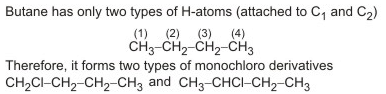VITEEE PCME Mock Test - 1 - Question 85

Which of the following reactions can be used for the synthesis of an alkene

VITEEE PCME Mock Test - 1 - Question 86

In the following reaction, which choice has value twice that of the equivalent mass of the oxidising agent SO₂ + H₂O → 3S + 2H₂O

VITEEE PCME Mock Test - 1 - Question 87

The significant figures in 3400 are

VITEEE PCME Mock Test - 1 - Question 88

The hormone which controls the processes of burning of fats, proteins and carbohydrates to librates energy in the body is

VITEEE PCME Mock Test - 1 - Question 89

The α-amino acids, usually, exist in the form of zwitter ions. It means they consist of

VITEEE PCME Mock Test - 1 - Question 90

On heating with conc. HNO₃, proteins give yellow colour. This test is called

Detailed Solution for VITEEE PCME Mock Test - 1 - Question 90 The xanthoproteic reaction is a method that can be used to determine the amount of protein soluble in a solution, using concentrated nitric acid. The test gives a positive result in amino acids carrying aromatic groups, especially in the presence of tyrosine.
VITEEE PCME Mock Test - 1 - Question 91

Calcium acetate when dry distilled gives

VITEEE PCME Mock Test - 1 - Question 92

Which of the following does not contain a carboxyl group?

VITEEE PCME Mock Test - 1 - Question 93

Toluic acid on reaction with Br₂ + Fe gives

VITEEE PCME Mock Test - 1 - Question 94

The reaction,SO₂ + Cl₂ → SO₂Cl₂ is exothermic and reversible.A mixture of SO₂(g),Cl₂(g) and SO₂Cl₂ (l) is at equilibrium in a closed container.Now a certain quantity of extra SO₂ is introduced into the container , the volume remaining the same.which of the following is/are true ?

VITEEE PCME Mock Test - 1 - Question 95

when a catalyst is added to a reversible reaction in equilibrium state, the value of equilibrium constant

VITEEE PCME Mock Test - 1 - Question 96

Heat of combustion ΔH for C(s), H2(g) and CH4(g) are -94, -68 and -213 cal/mol. Then ΔH for C(s) + 2H2(g) → CH4(g) is

Detailed Solution for VITEEE PCME Mock Test - 1 - Question 96

CH4 + 2O2 = CO2 + 2H2O
ΔH = -213 cal/mol .....(1)
C + O2 = CO2
ΔH = - 94 cal/mol .....(2)
H2 + (1/2) O2 = H2O
ΔH = - 68 cal/mol .....(3)
ΔH for C(s) + 2H2(g) → CH4(g)
= 2(eq 3) + (eq 2) - (eq 1)
= (- 68x2) + (- 94) - (- 213) = -17Kcal

VITEEE PCME Mock Test - 1 - Question 97

Internal energy of a substance/system is a

VITEEE PCME Mock Test - 1 - Question 98

Given C(s) + O₂(g) → CO₂(g) ; ∆H = −395kJ, S(s) + O₂(g) → SO₂(g);∆H = −295kJ , CS₂(l) + 3O₂(g) → CO₂(g) + 2SO₂(g) ; ∆H = −1110kJ. The heat of formation of CS₂(l) is

VITEEE PCME Mock Test - 1 - Question 99

Detergents are prepared by the action of H₂SO₄ followed by neutralization by starting with

VITEEE PCME Mock Test - 1 - Question 100

The IUPAC name of (CH₃)₂CHCH₃ is

VITEEE PCME Mock Test - 1 - Question 101

In which of the following complex ion, the central metal ion is in a state of sp3 d2 hybridisation?

VITEEE PCME Mock Test - 1 - Question 102

The reaction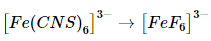takes place with

VITEEE PCME Mock Test - 1 - Question 103

Acetaldoxime reacts with P₂O₅ to give

VITEEE PCME Mock Test - 1 - Question 104

Dye test can be used to distinguish between

VITEEE PCME Mock Test - 1 - Question 105

Corrosion can be prevented by

VITEEE PCME Mock Test - 1 - Question 106

During the electrolysis of a solution of AgNO₃, 9650 C of charge passes through the electroplating bath, the mass of silver deposited on the cathode will be

VITEEE PCME Mock Test - 1 - Question 107

The standard reduction potentials of 4 elements are given below.Which of the following will be the most suitable reducing agent?
I = -3.04 V, II = -1.90 V, III =0 V, IV = 1.90 V

VITEEE PCME Mock Test - 1 - Question 108

Which of the following is simple ether ?

VITEEE PCME Mock Test - 1 - Question 109

The internal energy of one mole of an ideal gas is given by

VITEEE PCME Mock Test - 1 - Question 110

The most common alum is

VITEEE PCME Mock Test - 1 - Question 111

Which of the following is used in the preparation of aerated water (soda)?

VITEEE PCME Mock Test - 1 - Question 112

Which of the following compound is not known

VITEEE PCME Mock Test - 1 - Question 113

Which of the following contains a coordinate covalent bond ?

VITEEE PCME Mock Test - 1 - Question 114

In the conversion Br₂ → BrO₃- the oxidation state of bromine changes from

VITEEE PCME Mock Test - 1 - Question 115

The blue complex formed on addition of conc. NH₄OH solution to a Cu2+ salt solution has the structure?

VITEEE PCME Mock Test - 1 - Question 116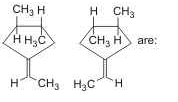VITEEE PCME Mock Test - 1 - Question 117

Which among the following statements is false?

VITEEE PCME Mock Test - 1 - Question 118

Space lattice of CaF₂ is

VITEEE PCME Mock Test - 1 - Question 119

Two moles of an aldehyde react with concentrated solution of sodium hydroxide and produce one mole of acid and one mole of alcohol. The aldehyde is

VITEEE PCME Mock Test - 1 - Question 120

During extraction of silver, which of the following is formed ?

VITEEE PCME Mock Test - 1 - Question 121

Find the antonym of CELIBATE

VITEEE PCME Mock Test - 1 - Question 122

Fill in the blank with appropriate word.
The miser gazed.........at the pile of gold coins in front of him.

VITEEE PCME Mock Test - 1 - Question 123

Find the synonym of AMENDABLE

VITEEE PCME Mock Test - 1 - Question 124

Out of the given alternatives, choose the one which can be substituted for the given capitalised word.

He does not like to be friendly with Sarita; he always GIVES HER A COLD SHOULDER.

VITEEE PCME Mock Test - 1 - Question 125

Improve the sentence by choosing best alternative for capitalised part of the sentence.

No one could explain how a calm and balanced person like him could PENETRATE such a mindless act on his friends.

## VITEEE: Subject Wise and Full Length MOCK Tests

1 videos|3 docs|73 tests
 Use Code STAYHOME200 and get INR 200 additional OFF Use Coupon Code
Information about VITEEE PCME Mock Test - 1 Page
In this test you can find the Exam questions for VITEEE PCME Mock Test - 1 solved & explained in the simplest way possible. Besides giving Questions and answers for VITEEE PCME Mock Test - 1, EduRev gives you an ample number of Online tests for practice

## VITEEE: Subject Wise and Full Length MOCK Tests

1 videos|3 docs|73 tests